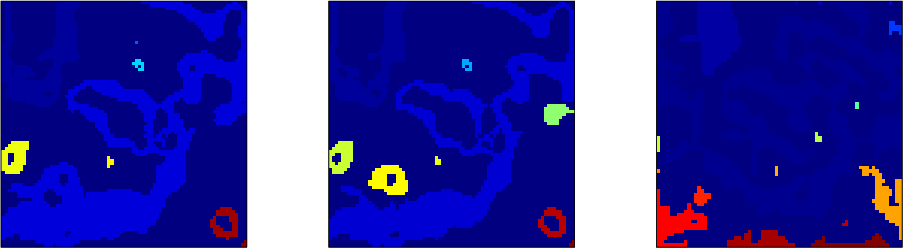## Tutorial on interpolation

November 25, 2019 Examples No comments

The example in rsf/tutorials/interp reproduces the tutorial from Matt Hall on interpolation methods.

The tutorial was published in the April 2016 issue of The Leading Edge.Madagascar users are encouraged to try improving the results.

## Tutorial on mapping lineaments

September 14, 2019 Examples No comments

The example in rsf/tutorials/lineaments reproduces the tutorial from Matteo Niccoli on mapping and validating lineaments.

The tutorial was published in the August 2015 issue of The Leading Edge.Madagascar users are encouraged to try improving the results.

## Plotting with matplotlib

September 12, 2019 Programs No comments

Matplotlib is a popular Python library for 2D plotting, which emulates (and exceeds) the plotting functionality of MATLAB.

At the Madagascar Working Workshop in 2018, a couple of new scripts were added to simplify plotting with Matplotlib in Madagascar.

• sfmatplotlib can be used to plot RSF files.You can use it as follows:
sfmatplotlib <matplotlib function> <plot options> [format=eps] < inp.rsf [ > out.eps]


Without the standard output, the figure is displayed on the screen.The figure above was generated with

sfspike n1=1000 k1=300 | sfbandpass fhi=2 phase=y| sfmatplotlib plot title="Welcome to Maagascar" grid=y linewidth=3 format=png > figure.png


Futher enhancements are possible.

• sfpgreywfl is a script contributed by Carlos da Costa that uses matplotlib for plotting a seismic wavefiels overlaid on the velocity model.The figure above was generated with

sfpgreywfl < wavefield.rsf bg=velocity.rsf wflcmap=seismic title="Wavefield" barlabel="Velocity (m/s)" tmin=0.08 savefile=figure.gif


September 9, 2019 Celebration No comments

The major version of Madagascar, stable version 3.0, has been released. The main change is the added support for Python-3. Both Python-2 and Python-3 are now supported. The new version also features 14 new reproducible papers, as well as other enhancements.

According to the SourceForge statistics, the previous 2.0 stable distribution has been downloaded about 6,000 times. The top country (with 27% of all downloads) was China, followed by the USA, Brazil, Canada, and India.

In September 2019, the total cumulative number of downloads for the stable version of Madagascar has reached 50 thousand. The current development version continues to be available through Github.## scons2jupyter

September 9, 2019 Programs No comments

scons2jupyter is a simple script that converts a Madagascar-style SConstruct file to a Jupyter notebook with a Python interface.

You can use it by simply running

scons2jupyter < SConstruct > mynotebook.ipynb


An example notebook is the result of running scons2jupyter in bei/vela/vscan.A newly created notebook can be populated with Markdown text and explanations to implement the discipline of literate programmins.

## Program of the month: sfsnr2

August 10, 2019 Programs No comments

sfsnr2 computes the signal-to-noise (SNR) ratio in decibells.

The following example from tccs/intshape/fsyn uses the SNR computation to compare the convergence of two different methods.SNR is computed according to the equation

$SNR=\displaystyle 10\log_{10}\left(\frac{\Arrowvert \mathbf{s} \Arrowvert_2^2}{\Arrowvert\mathbf{n}\Arrowvert_2^2}\right)$

The signal vector $\mathbf{s}$ is in the standard input, the noise vector $\mathbf{n}$ is in the file specified by noise= parameter.

## Low-rank viscoacoustic wave extrapolation

July 18, 2019 Documentation No comments

A new paper is added to the collection of reproducible documents: Viscoacoustic modeling and imaging using low-rank approximationA constant-$Q$ wave equation involving fractional Laplacians was recently introduced for viscoacoustic modeling and imaging. This fractional wave equation has a convenient mixed-domain space-wavenumber formulation, which involves the fractional-Laplacian operators with a spatially varying power. We propose to apply low-rank approximation to the mixed-domain symbol, which enables a space-variable attenuation specified by the variable fractional power of the Laplacians. Using the proposed approximation scheme, we formulate the framework of the $Q$-compensated reverse-time migration ($Q$-RTM) for attenuation compensation. Numerical examples using synthetic data demonstrate the improved accuracy of using low-rank wave extrapolation with a constant-$Q$ fractional-Laplacian wave equation for seismic modeling and migration in attenuating media. Low-rank $Q$-RTM applied to viscoacoustic data is capable of producing images comparable in quality with those produced by conventional RTM from acoustic data.

## Program of the month: sflpf

July 9, 2019 Programs No comments

sfslpf estimates a non-stationary filter using shaping regularization.

The method is described in the reproducible paper Adaptive multiple subtraction using regularized nonstationary regressio

The following example from tccs/lpf/plut shows a common-offset section from the Pluto synthetic dataset before and after adaptive multiple subtraction with the help of sflpf.Given target data $m(\mathbf{x})$ (specified with match= parameter) and a collection of fitting functions $s_k(\mathbf{x})$ (specified in the standard input), sflpf finds the fitting coefficients $b_k(\mathbf{x})$ by minimizing the error

$m(\mathbf{x}) – \displaystyle \sum_{k=1}^{N} b_k(\mathbf{x})\,s_k(\mathbf{x})$

while constraining the coefficients to be smooth. The smoothness is controlled by rect#= parameters, as in sfsmooth.

Shaping regularization is carried out iteratively, niter= controls the number of iterations.

The mean coefficient from the example above is shown in the figure below.Optionally, a prediction-error filter can be applied to whiten the residual. The filter is specified with the help of pef= and lag= parameters, with a multidimensional helical filter specified as in sfhelicon.

The complex version of the same program is sfclpf.

## Program of the month: sfslice

June 12, 2019 Programs No comments

sfslice extracts a slice from the data given by a curve (in 2-D) or a surface (in 3-D).

The right plot in the following example from gee/prc/bob shows the semblance extracted using sfslice from the semblance scan along the trajectory picked with sfpick.sfslice does not have parameters other than pick=, which points to the file with the surface for extraction.

It performs a simple linear interpolation to extract the values.

## sfbargraph

June 6, 2019 Programs No comments

sfbargraph is a new plotting program for displaying data as bars.

The bottom plot in the figure below shows a simple example from rsf/rsf/sfnoise.The unique parameters of sfbargraph include width= for controling the relative width of the bars and stack= to control if the bars from multiple input traces should be stacked on top of one another.

The other parameters are shared with other plotting programs.